# Introduction

Tracking data can be difficult to analyze because of different errors and artifacts that can occur in the data and cause bias in the analysis. This vignette will explain how the package can be used to detect and correct for some of these errors. The topics will be:

1. Checking track lengths and dealing with short tracks
2. Detecting and correcting tissue drift
3. Detecting and correcting tracking errors and imaging artifacts using angle analyses
4. Detecting and correcting variation in timesteps

# Dataset

First load the package:

library( celltrackR )
library( ggplot2 )


The package contains a dataset of T cells imaged in a mouse peripheral lymph node using two photon microscopy. We will here use this dataset as an example of how to perform quality control.

The dataset consists of 21 tracks of individual cells in a tracks object:

str( TCells, list.len = 3 )

## List of 22
##  $0 : num [1:11, 1:4] 0 27.8 55.5 83.3 111.1 ... ## ..- attr(*, "dimnames")=List of 2 ## .. ..$ : chr [1:11] "1" "2" "3" "4" ...
##   .. ..$: chr [1:4] "t" "x" "y" "z" ##$ 1 : num [1:11, 1:4] 0 27.8 55.5 83.3 111.1 ...
##   ..- attr(*, "dimnames")=List of 2
##   .. ..$: chr [1:11] "1" "2" "3" "4" ... ## .. ..$ : chr [1:4] "t" "x" "y" "z"
##  $2 : num [1:39, 1:4] 0 27.8 55.5 83.3 111.1 ... ## ..- attr(*, "dimnames")=List of 2 ## .. ..$ : chr [1:39] "1" "2" "3" "4" ...
df$id[ !(df$angle < angle.thresh & df$dist < dist.thresh) ] <- "" # Plot ggplot( df, aes( x = dist, y = angle ) ) + geom_point( color = "gray40" ) + geom_text( aes( label = id ), color = "red" ) + labs( x = "distance between cell pairs", y = "angle between cell pairs" ) + geom_hline( yintercept = angle.thresh, col = "blue",lty=2 ) + geom_vline( xintercept = dist.thresh, col = "blue", lty=2) + theme_classic()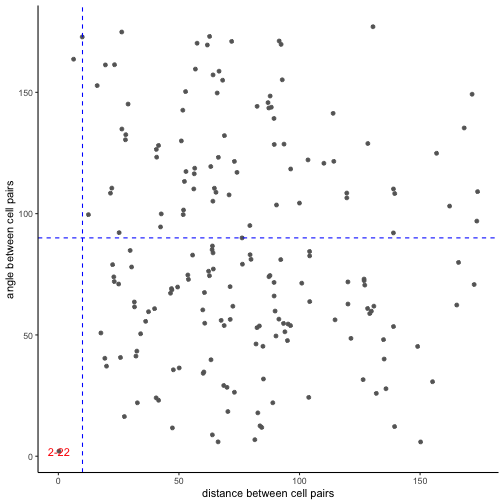We indeed find the pair of tracks with ids “2” (the original track) and “22” (the noisy duplicate of the original track). Plot these tracks specifically to check: plot( TCells.dup[c("2","22")])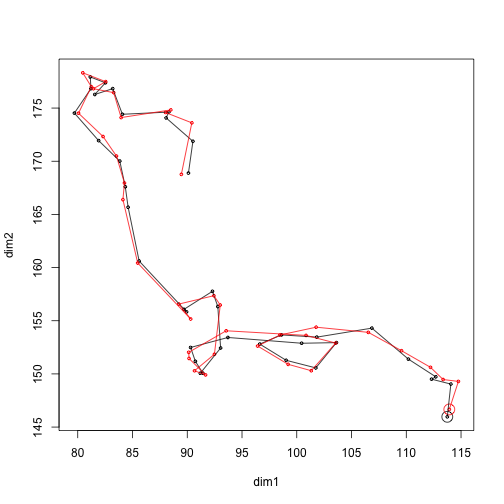That indeed looks like double tracking. ### 3.2 Detecting tracking errors near border or imprecise z-calibration: distances and angles to border planes A plot of angles and distances to the border planes of the imaging volume can help detect artifacts (Beltman et al (2009) ). We can compute these for each step using the functions distanceToPlane and angleToPlane. To specify a border plane, we first need three points on that plane. We can either define the imaging volume manually or estimate it using boundingBox: tracks <- TCells bb <- boundingBox( tracks ) bb  ## t x y z ## min 0.00 26.675 17.2098 1.25 ## max 1056.11 215.526 228.7770 51.25  Let's take the two borders in the z-dimension as an example. The lower z-plane contains points (minx,miny,minz), (maxx,miny,minz), (maxx,maxy,minz). The upper z-plane is at distance (maxz-minz) from the lower plane. # Define points: lower1 <- c( bb["min","x"], bb["min","y"], bb["min","z"] ) lower2 <- c( bb["max","x"], bb["min","y"], bb["min","z"] ) lower3 <- c( bb["max","x"], bb["max","y"], bb["min","z"] ) zsize <- bb["max","z"] - bb["min","z"] # Compute angles and distances of steps to this plane. single.steps <- subtracks( tracks, 1 ) angles <- sapply( single.steps, angleToPlane, p1 = lower1, p2 = lower2, p3 = lower3 ) distances <- sapply( single.steps, distanceToPlane, p1 = lower1, p2 = lower2, p3 = lower3 ) df <- data.frame( angles = angles, distances = distances ) # Plot ggplot( df, aes( x = distances, y = angles ) ) + geom_point( color = "gray40" ) + stat_smooth( method = "loess", span = 1, color = "black" ) + geom_hline( yintercept = 32.7, color = "red" ) + scale_x_continuous( limits=c(0,zsize ), expand = c(0,0) ) + theme_classic()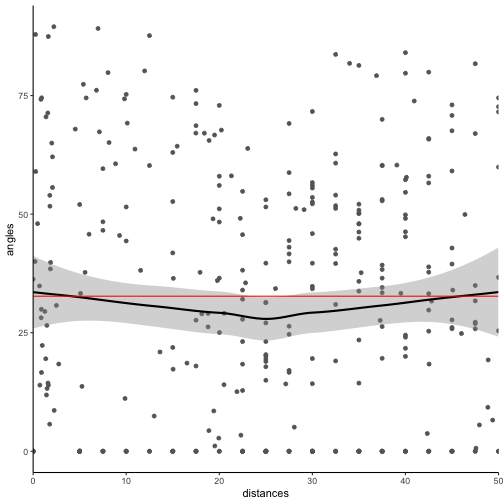The mean angle to the border plane should be roughly 32.7 degrees, and indeed this seems to be the case. Tracking errors near the border plane would result in a lower average angle near the left and right side of the plots, whereas imprecise z-calibration would result in a systematic deviation from the 32.7 degree angle at any distance to the plane (Beltman et al (2009) ). # 4 Detecting and correcting variation in time resolution ### 4.1 Detecting variation in timesteps Track analysis methods usually assume a constant time interval $$\Delta t$$ between consecutive images in a time-lapse microscopy dataset. In reality, there are mostly at least small fluctuations in the $$\Delta t$$ between consecutive images. To find the $$\Delta t$$ of each step, we first extract single steps using the subtracks() function (see also the vignette on analysis methods): # Extract all subtracks of length 1 (that is, all "steps") single.steps <- subtracks( TCells, 1 ) # The output is a new tracks object with a unique track for each step # in the data (no longer grouped by the original cell they came from): str( single.steps, list.len = 3 )  ## List of 359 ##$ 0.1  : num [1:2, 1:4] 0 27.8 132.5 133.9 118.7 ...
##   ..- attr(*, "dimnames")=List of 2
##   .. ..$: chr [1:2] "1" "2" ## .. ..$ : chr [1:4] "t" "x" "y" "z"
##  $0.2 : num [1:2, 1:4] 27.8 55.5 133.9 131.8 118.7 ... ## ..- attr(*, "dimnames")=List of 2 ## .. ..$ : chr [1:2] "2" "3"
##   .. ..$: chr [1:4] "t" "x" "y" "z" ##$ 0.3  : num [1:2, 1:4] 55.5 83.3 131.8 133.2 118.1 ...
##   ..- attr(*, "dimnames")=List of 2
##   .. ..$: chr [1:2] "3" "4" ## .. ..$ : chr [1:4] "t" "x" "y" "z"
##   [list output truncated]
##  - attr(*, "class")= chr "tracks"


After extracting the single steps, we can find the median $$\Delta t$$ using the timeStep() function:

median.dt <- timeStep( TCells )
median.dt

##  27.797


We then find the actual $$\Delta t$$ of each step by applying the duration() function to each step in the single.steps object:

step.dt <- sapply( single.steps, duration )
str(step.dt)

##  Named num [1:359] 27.8 27.7 27.8 27.8 27.8 ...
##  - attr(*, "names")= chr [1:359] "0.1" "0.2" "0.3" "0.4" ...


And visualize the difference with the median $$\Delta t$$ in a histogram (expressed as a percentage of $$\Delta t$$:

dt.diff.perc <- (step.dt - median.dt) * 100 / median.dt
hist( dt.diff.perc, xlab = "dt (percentage difference from median)" )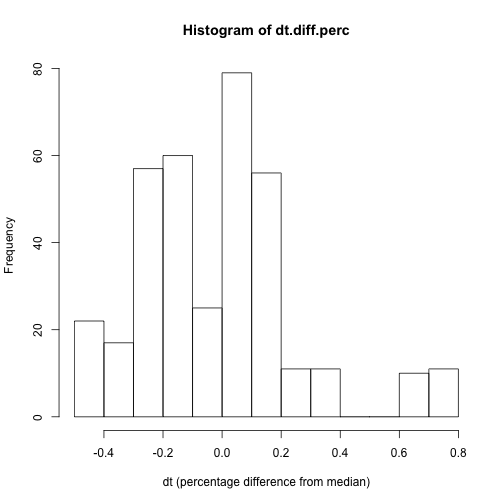Thus, the TCell dataset indeed contains fluctuations in $$\Delta t$$, but they are small: none are more than 1 percent of the median $$\Delta t$$ in the data.

### 4.2 Example: detecting missing data in tracks

As an example of a dataset containing gaps in tracks, we will introduce an artificial error by removing some timepoints in a few tracks in the TCell data:

# This function randomly removes coordinates from a track dataset with probability "prob"
remove.points <- function( track, prob=0.1 ){

tlength <- nrow( track )
remove.rows <- sample( c(TRUE,FALSE), tlength, replace=TRUE,
prob = c(prob, (1-prob) ) )
track <- track[!remove.rows,]
return(track)
}

# Apply function to dataset to randomly remove coordinates in the data
TCells.gap <- as.tracks( lapply( TCells, remove.points ) )


Create the same histogram as before:

# median dt of the new data
median.dt.gap <- timeStep( TCells.gap )

# duration of the individual steps
steps.gap <- subtracks( TCells.gap, 1 )
step.dt.gap <- sapply( steps.gap, duration )

# express difference as percentage of median dt
dt.diff.perc.gap <- (step.dt.gap - median.dt.gap) * 100 / median.dt.gap
hist( dt.diff.perc.gap, xlab = "dt (percentage difference from median)" )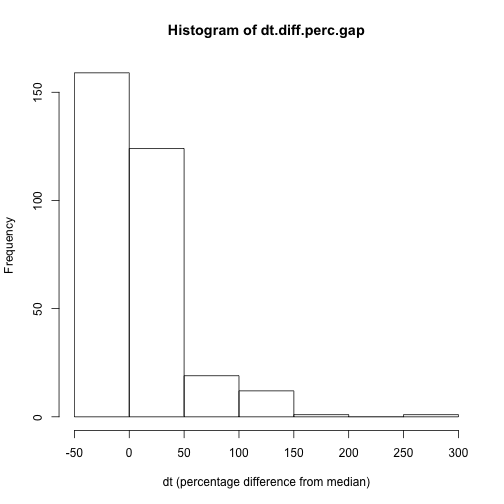Now, the percentage difference with the median $$\Delta t$$ is often much larger.

This has an effect on for example the step-based displacement:

T1.step.disp <- sapply( single.steps, displacement )
T1.gap.disp <- sapply( steps.gap, displacement )

lapply( list( original = T1.step.disp, gaps = T1.gap.disp ), summary )

## $original ## Min. 1st Qu. Median Mean 3rd Qu. Max. ## 0.211 2.050 4.017 4.432 6.282 18.487 ## ##$gaps
##    Min. 1st Qu.  Median    Mean 3rd Qu.    Max.
##   0.211   2.387   4.237   4.774   6.539  19.095


Note that the mean and median displacement are slightly higher due to the gaps, because steps with a gap in between are actually two steps and thus have a larger displacement.

For a simple step-based analysis of displacement, we can use normalizeToDuration() to correct for differences in time resolution:

T1.norm.disp <- sapply( single.steps, normalizeToDuration( displacement ) )
T1.norm.gap.disp <- sapply( steps.gap, normalizeToDuration( displacement ) )
lapply( list( original = T1.norm.disp, gaps = T1.norm.gap.disp ), summary )

## $original ## Min. 1st Qu. Median Mean 3rd Qu. Max. ## 0.007591 0.073433 0.144298 0.159449 0.226024 0.664722 ## ##$gaps
##     Min.  1st Qu.   Median     Mean  3rd Qu.     Max.
## 0.004189 0.072886 0.144560 0.157534 0.222115 0.664722


The difference in displacements is now gone. For more complicated analyses, one might want to correct the gaps in the dataset via splitting tracks or via interpolation – see the next section.

### 4.3 Correcting gaps or variation in timesteps

If a dataset contains gaps, as detected in the histogram of $$\Delta t$$, we can correct these using the repairGaps function, where we can select one of three correction methods using the how argument:

1. Dropping all tracks with gaps (how = "drop")
2. Splitting tracks around the gaps(how = "split")
3. Interpolating the track (how = "interpolate")

For example:

# Repair gaps by splitting or interpolation, the number of tracks is
# different after each fix
split.gap <- repairGaps( TCells.gap, how = "split" )
interpolate.gap <- repairGaps( TCells.gap, how = "interpolate" )

c( "after splitting" = length( split.gap),
"after interpolation" = length( interpolate.gap ) )

##     after splitting after interpolation
##                  46                  22


### 4.4 Comparing experiments with a different time resolution

Finally, if we wish to compare tracks from experiments imaged at a different time resolution $$\Delta t$$, we can use the function interpolateTrack() to estimate the position of each cell at any set of timepoints of interest.

For example, let's create a second T cell dataset where we keep only every second timepoint (to effectively increase $$\Delta t$$ twofold while keeping the same dataset):

T2 <- subsample( TCells, k = 2 )


When we now perform a step-based analysis of displacement, the two will be different:

# displacement
T1.steps <- subtracks( TCells, 1 )
T1.disp <- sapply( T1.steps, displacement )

T2.steps <- subtracks( T2, 1 )
T2.disp <- sapply( T2.steps, displacement )

lapply( list( T1 = T1.disp, T2 = T2.disp ), summary )

## $T1 ## Min. 1st Qu. Median Mean 3rd Qu. Max. ## 0.211 2.050 4.017 4.432 6.282 18.487 ## ##$T2
##    Min. 1st Qu.  Median    Mean 3rd Qu.    Max.
##   0.233   3.458   7.841   7.739  10.832  20.923


To correct for the difference in time resolution, we can interpolate both datasets at a fixed time resolution:

# interpolate both datasets at the time resolution of the neutrophils
dt <- timeStep( TCells )
interpolate.dt <- function( x, dt, how = "spline" ){
trange <- range( timePoints( wrapTrack( x ) ) )
tvec <- seq( trange, trange, by = dt )
x <- interpolateTrack( x, tvec, how = how )
return(x)
}

T1.corrected <- as.tracks( lapply( TCells, interpolate.dt, dt = dt ) )
T2.corrected <- as.tracks( lapply( T2, interpolate.dt, dt = dt ) )

# Check the effect on the displacement statistics:
T1.corr.steps <- subtracks( T1.corrected, 1 )
T1.corr.disp <- sapply( T1.corr.steps, displacement )

T2.corr.steps <- subtracks( T2.corrected, 1 )
T2.corr.disp <- sapply( T2.corr.steps, displacement )

lapply( list( T1 = T1.disp, T2 = T2.disp,
T1.corr = T1.corr.disp, T2.corr = T2.corr.disp ),
summary )

## $T1 ## Min. 1st Qu. Median Mean 3rd Qu. Max. ## 0.211 2.050 4.017 4.432 6.282 18.487 ## ##$T2
##    Min. 1st Qu.  Median    Mean 3rd Qu.    Max.
##   0.233   3.458   7.841   7.739  10.832  20.923
##
## $T1.corr ## Min. 1st Qu. Median Mean 3rd Qu. Max. ## 0.2127 2.0729 4.0147 4.3814 6.2596 13.2353 ## ##$T2.corr
##    Min. 1st Qu.  Median    Mean 3rd Qu.    Max.
##  0.1329  1.9222  3.9703  3.9432  5.3959 12.2322


The difference is now much smaller.